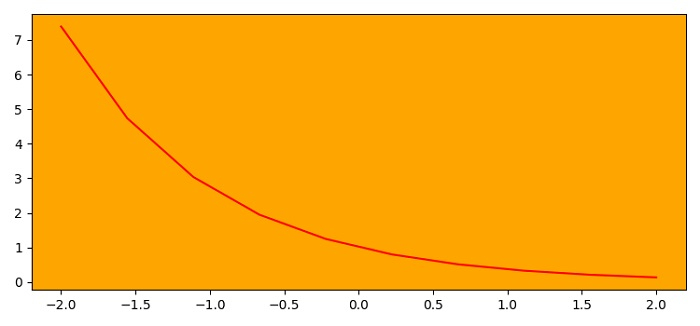# How to change axes background color in Matplotlib?

To change the axes background color, we can use set_facecolor() method.

## Steps

• Set the figure size and adjust the padding between and around the subplots.

• Get the current axes using gca() method.

• Set the facecolor of the axes.

• Create x and y data points using numpy.

• Plot x and y data points using plot() method.

• To display the figure, use show() method.

## Example

import numpy as np
import matplotlib.pyplot as plt

plt.rcParams["figure.figsize"] = [7.50, 3.50]
plt.rcParams["figure.autolayout"] = True

ax = plt.gca()
ax.set_facecolor("orange")

x = np.linspace(-2, 2, 10)
y = np.exp(-x)

plt.plot(x, y, color='red')

plt.show()

## Output